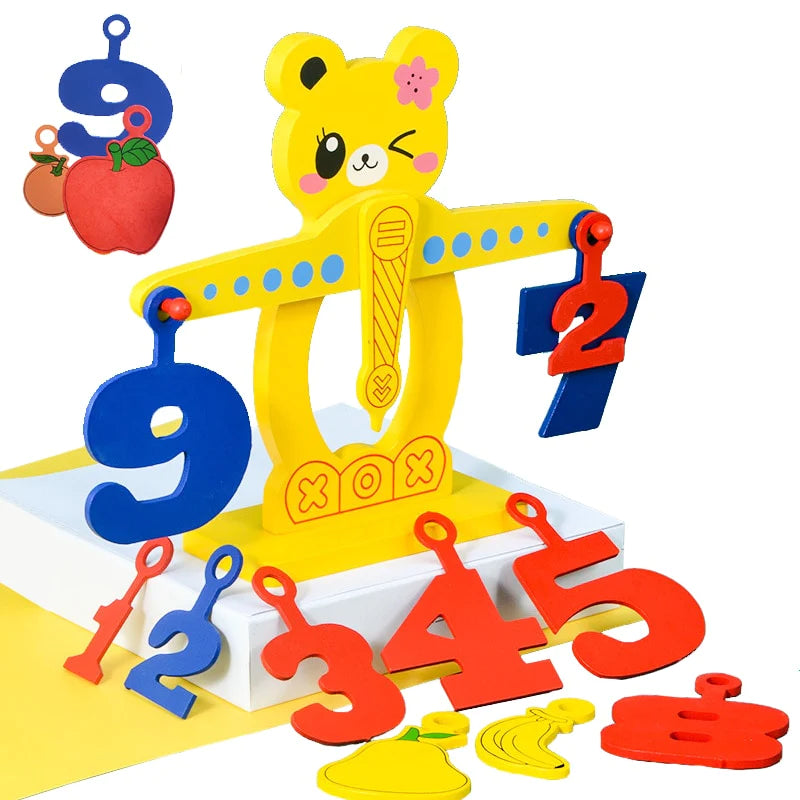# Math Balance Scales: Why Everyone is Embracing this Educational Tool

In the world of education, some tools have the power to captivate the hearts and minds of both educators and students. The math balance scale is one such tool that is gaining increasing popularity for its ability to make learning mathematics engaging and intuitive. This article will explore the features of a math balance scale, its educational significance, and why it's becoming a must-have tool in classrooms and homes.

## The Symbolic Meaning of a Math Balance Scale

Much like the cactus symbolizes endurance, the math balance scale symbolizes balance and equality. It represents the fundamental principles of mathematics, where both sides of an equation must be in equilibrium. By using a balance scale, children learn the concept of balance in equations, setting a strong foundation for mathematical understanding.

## Why Choose a Math Balance Scale?

The math balance scale is a favorite educational tool for its ability to simplify complex mathematical concepts and make learning fun. Here are some compelling reasons why it's gaining popularity:

Hands-On Learning

Math balance scales provide a tactile and visual way for students to grasp abstract mathematical concepts. They can physically see the balance or imbalance of equations, making math less intimidating.

Material Quality

High-quality math balance scales are made from durable materials that can withstand the rigors of classroom or home use. They are designed to be safe for children and are built to last.

Versatility

These scales are versatile tools that can be used to teach a wide range of math concepts, from basic arithmetic to more advanced algebraic equations. They grow with the child's mathematical abilities.

Interactive Learning

Math balance scales encourage active participation. Students can experiment with different weights and numbers, fostering a deeper understanding of mathematical relationships.

## Educational Applications

Math balance scales have a wide range of applications in education:

Basic Arithmetic

For young learners, they serve as a visual aid for teaching addition, subtraction, multiplication, and division. Balancing equations helps students understand the concept of equality.

Algebraic Concepts

As students progress, math balance scales can be used to teach algebraic equations, inequalities, and even concepts like solving for variables.

Problem Solving

They are valuable tools for teaching problem-solving skills, as students can use the scales to work through complex math problems step by step.

Group Activities

In classrooms, math balance scales can be used in group activities, promoting collaborative learning and discussions around mathematical concepts.

## Who Can Benefit?

Math balance scales are suitable for a wide range of learners:

Elementary Students

These scales can be introduced in the early years to build a strong foundation in mathematics.

Middle and High School Students

They are valuable tools for reinforcing mathematical concepts and preparing for more advanced math courses.

Homeschooling Parents

Math balance scales are excellent additions to homeschooling curricula, providing hands-on learning opportunities.

## In Conclusion

Much like the cactus plush toy, which symbolizes resilience and endurance, the math balance scale symbolizes balance and equality in the realm of education. By incorporating this tool into learning, educators and parents empower children to embrace mathematics with confidence. Its hands-on approach transforms math from a daunting subject into an exciting journey of discovery. Whether in a classroom or a home learning environment, the math balance scale is becoming an essential companion on the path to mathematical mastery. You can find one at educational supply stores or explore online options to bring this valuable tool into your child's learning experience.